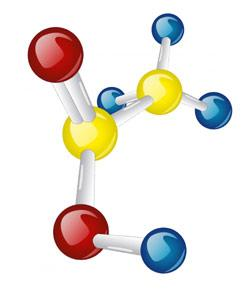Mrs. Lawrence's Covalent Bonding Quiz

8 QuestionsSettings.

Related Topics
• 1.
Which statement is TRUE?
• A.

A double bond shares three pairs of electrons.

• B.

Resonance is when a molecule has more than one possible Lewis dot structure.

• C.

An electronegativity difference of 1.5 between 2 atoms results in a nonpolar covalent bond.

• D.

Polar covalent bonds result when valence electrons are shared equally between 2 atoms.

• 2.
What is the name of NO?
• A.

Nitrogen oxide

• B.

Dinitrogen oxide

• C.

Nitrogen monoxide

• D.

Mononitrogen monoxide

• 3.
What type of bond is formed between hydrogen and nitrogen? (electronegativity values: H = 2.1 and N = 3.0)
• A.

Nonpolar covalent

• B.

Metallic

• C.

Polar covalent

• D.

Ionic

• 4.
Which of the following forms a polar molecule?Hint: Draw the Lewis dot structure, determine the bond type, then determine the shape.eN values:  H = 2.1, S = 2.5, O = 3.5, Br = 2.8, I = 2.5, N = 3.0, C = 2.5, F = 4.0
• A.

Hydrogen iodide

• B.

Sulfur dioxide

• C.

Nitrogen tribromide

• D.

Carbon tetrafluoride

• 5.
What type of intermolecular force exists between the hydrogen of one water molecule and the oxygen of another?
• A.

Dispersion force

• B.

Van der Waals force

• C.

Dipole-dipole

• D.

Hydrogen bond

• 6.
Which of the following is an exception to the Octet Rule?
• A.

Hydrogen

• B.

Carbon

• C.

Nitrogen

• D.

Oxygen

• 7.
Which of the following forms four covalent bonds?
• A.

Hydrogen

• B.

Carbon

• C.

Nitrogen

• D.

Sulfur

• 8.
Which of the following is the molecular shape of ammonia?
• A.

Linear

• B.

Bent

• C.

Pyramidal

• D.

Tetrahedral